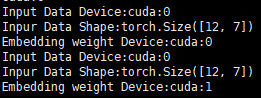# DataParallel can not split data to different gpus

My pytorch model can run on a single gpu correctly. But when I use DataParallel to run it on multiple gpus ,I got an error : arguments are located on different GPUs

It seems that embedding parameters and input tensor are on different gpu. So I print the divice of them:The batch size is 12 and gpu num is 2. As is shown above, it has two problem:

1. The batch size should be 6 instead of 12 (cause it should be split into two pices by DataParallel).
2. The embedding weigh are parallel to two gpus(cuda:0 and cuda:1) but the input data on the same gpu.

I use torchtext to load data and the input data is on the cuda:0.
I use the following code to parallelize model:

``````    self.device, device_ids = self._prepare_device(config['n_gpu'])
self.model = model.to(self.device)
# data parrallel
if len(device_ids) > 1:
self.model = torch.nn.DataParallel(model, device_ids=device_ids)
...
def _prepare_device(self, n_gpu_use):
"""
setup GPU device if available, move model into configured device
"""
n_gpu = torch.cuda.device_count()
if n_gpu_use > 0 and n_gpu == 0:
self.logger.warning(
"Warning: There\'s no GPU available on this machine, training will be performed on CPU.")
n_gpu_use = 0
if n_gpu_use > n_gpu:
msg = "Warning: The number of GPU\'s configured to use is {}, but only {} are available on this machine.".format(
n_gpu_use, n_gpu)
self.logger.warning(msg)
n_gpu_use = n_gpu
device = torch.device('cuda:0' if n_gpu_use > 0 else 'cpu')
list_ids = list(range(n_gpu_use))
return device, list_ids
``````

And I use the completely same logic to parallelize another model and it works!

Could you post some code of your training loop?
Does your data contain another dummy dimension in `dim0`?

Thanks for reply. I have fix this problem by changing the model input. Previously, I directly put torchtext iterator object into the model:

``````for batch_idx, batch in enumerate(self.data_loader.train_iter):
output = self.model(batch)
``````

Then I modified the code and it works：

``````for batch_idx, batch in enumerate(self.data_loader.train_iter):
input_data = {
'q_word': batch.q_word,
'q_lens': batch.q_word,
'paras_word': batch.paras_word,
'paras_num': batch.paras_word,
'paras_lens': batch.paras_word,
}
output = self.model(input_data)
``````

Or like this:

``````for batch_idx, batch in enumerate(self.data_loader.train_iter):
output = self.model(batch.q_word, batch.q_word, \
batch.paras_word, batch.paras_word, batch.paras_word)
``````

All the value of the `input_data` are tensor.
I think the key problem is that you should feed tensor or a dic of tensors so that the DataParallel model can find the tensor and split the dimension in `dim0`.
I think that DataParallel should at least gives a warninig when the DataParallel can’t find any tensor that can be split in `dim0`

2 Likes